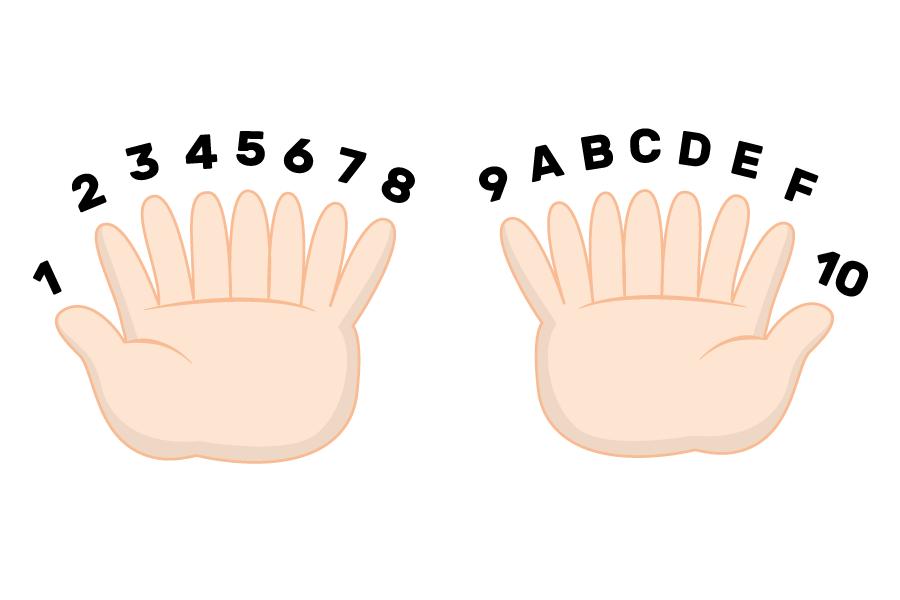## Want to keep learning?

This content is taken from the Raspberry Pi Foundation & National Centre for Computing Education's online course, Representing Data with Images and Sound: Bringing Data to Life. Join the course to learn more.
1.10

## Raspberry Pi Foundation

Now you’ll learn the basics of the number system known as hexadecimal: I’ll give you an overview of the digits used to represent hexadecimal numbers, as well as how to convert numbers into hexadecimal from denary or binary.

Which of the following numbers is easier to remember?

• 1100 0101
• C5

If you think C5 is easier and more convenient to remember and use, you now understand why some binary is expressed in hexadecimal format. Also, as we discussed earlier this week, technology has become very advanced using 1s and 0s, and this is partially because computers can represent very long pieces of data with shorter ones. C5 is actually the same value as 1100 0101, however it is represented as hexadecimal instead of binary.

In previous steps, I explained how different number systems work. Hexadecimal is a base 16 number system: it makes use of 16 numbers. So if we had a creature with 16 fingers, this is how they would count using hexadecimal:This table shows equivalent denary, binary, and hexadecimal values:

Denary Binary Hex
0 0000 0
1 0001 1
2 0010 2
3 0011 3
4 0100 4
5 0101 5
6 0110 6
7 0111 7
8 1000 8
9 1001 9
10 1010 A
11 1011 B
12 1100 C
13 1101 D
14 1110 E
15 1111 F

Hexadecimal is commonly used by computers because it can represent a byte of data with just two characters (instead of eight). Each hexadecimal character represents half a byte, also called a nibble. As hexadecimal is easier for humans to read than binary but is still easy to convert to binary, hexadecimal has a variety of uses, including in error messages, MAC addresses (used to identify a device on a network), character encoding, and RGB colours.

## Converting between hexadecimal and binary

Converting between hexadecimal and binary is straightforward: each nibble of binary (half a byte/four bits) corresponds to one hexadecimal character. You can use the table above to convert a hexadecimal symbol to four binary bits, or vice versa.

Going by the table above, the hexadecimal number 7D is converted to binary like this:

• 7 is equivalent to 0111 (the binary representation of the denary number 7)
• D is equivalent to 1101 (the binary representation of the denary number 13, which is represented in hexadecimal as D)
• So hexadecimal 7D is equivalent to binary 0111 1101

Simiarly, the binary number 0001 1010 is converted to hexadecimal like this:

• 0001 is the binary representation of 1 (which is the same in denary and hexadecimal)
• 1010 is the binary representation of the denary number 10, which is represented in hexadecimal as A
• So binary 0001 1010 is equivalent to hexadecimal 1A

## Converting hexadecimal to denary, via binary

You already know how to convert binary numbers to denary, and since you just learned how to convert a hexadecimal number to binary, you can also convert it to denary.

For example, the number A9 in hexadecimal is 1010 1001 in binary. And we can convert this to denary using the table we’ve used before:

128 64 32 16 8 4 2 1
1 0 1 0 1 0 0 1

128 + 32 + 8 + 1 = 169

## Converting hexadecimal directly to denary

We can also convert hexadecimal directly into denary by thinking about the place values. You know that the place values of denary numbers work like this:

1089 = (1 × 1000) + (0 × 100) + (8 × 10) + (9 × 1)

Base 10 place values 1000(10**3) 100(10**2) 10(10**1) 1 (10**0)
Denary value 1 0 8 9

Hexadecimal works very similarly, and with base 16 instead of base 10. Let’s convert hexadecimal A9 to denary:

Base 16 place values 16 (16**1) 1 (16**0)
• From the table above, we know that hexadecimal A represents denary 10 and hexadecimal 9 represents denary 9
• Just like in 1089, there is a 9 in the 1 position: (9 × 1)
• There is a 10 in the 16 position: (10×16)
• So that means hexadecimal A9 = (10 × 16) + (9 × 1) = 169 in denary.
Base 16 place values 16 (16**1) 1 (16**0)
• 200 / 16 = 12, remainder 8
• So denary 200 = (12 × 16) + (8 × 1) = C8 in hexadecimal